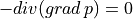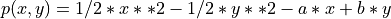# dg/laplace_2D.py¶

Description

Laplace equation solved in 2d by discontinous Galerkin methodon rectangle

p = 0 p_y = 0

[0,b]—————————–[a, b]

p_x = -a | p(x,y) | p_x = 0 p = 0 | | p = 0

[0,0]—————————–[a, 0]

p_y = b p = 0

solution to this is## Usage Examples¶

Run:

```sfepy-run sfepy/examples/dg/laplace_2D.py
```

Results are saved to output/dg/laplace_2D folder by default as `.msh` files, the best way to view them is through GMSH (http://gmsh.info/) version 4.6 or newer. Start GMSH and use `File | Open` menu or Crtl + O shortcut, navigate to the output folder, select all `.msh` files and hit Open, all files should load as one item in Post-processing named p_cell_nodes.

GMSH is capable of rendering high order approximations in individual elements, to modify fidelity of rendering, double click the displayed mesh, quick options menu should pop up, click on `All view options...`. This brings up the Options window with `View ` selected in left column. Under the tab `General` ensure that `Adapt visualization grid` is ticked, then you can adjust `Maximum recursion depth` and ``Target visualization error` to tune the visualization. To see visualization elements (as opposed to mesh elements) go to `Visibility` tab and tick `Draw element outlines`, this option is also available from quick options menu as `View element outlines` or under shortcut `Alt+E`. In the quick options menu, you can also modify normal raise by clicking `View Normal Raise` to see solution rendered as surface above the mesh. Note that for triangular meshes normal raise -1 produces expected raise above the mesh. This is due to the opposite orientation of the reference elements in GMSH and Sfepy and might get patched in the future.

`source code`

```r"""
Laplace equation solved in 2d by discontinous Galerkin method

.. math:: - div(grad\,p) = 0

on rectangle
p = 0
p_y = 0
[0,b]-----------------------------[a, b]
|                           |
|                           |
p_x = -a |         p(x,y)            | p_x = 0
p = 0    |                           | p = 0
|                           |
[0,0]-----------------------------[a, 0]
p_y = b
p = 0

solution to this is
.. math:: p(x,y) = 1/2*x**2 - 1/2*y**2 - a*x + b*y

Usage Examples
--------------

Run::

sfepy-run sfepy/examples/dg/laplace_2D.py

Results are saved to output/dg/laplace_2D folder by default as ``.msh`` files,
the best way to view them is through GMSH (http://gmsh.info/) version 4.6 or
newer. Start GMSH and use ``File | Open`` menu or Crtl + O shortcut, navigate to
the output folder, select all ``.msh`` files and hit Open, all files should load
as one item in Post-processing named p_cell_nodes.

GMSH is capable of rendering high order approximations in individual elements,
to modify fidelity of rendering, double click the displayed mesh, quick options
menu should pop up, click on ``All view options...``. This brings up the Options
window with ``View `` selected in left column. Under the tab ``General``
``Maximum recursion depth`` and ```Target visualization error`` to tune
the visualization. To see visualization elements (as opposed to mesh elements)
go to ``Visibility`` tab and tick ``Draw element outlines``, this option is also
available from quick options menu as ``View element outlines`` or under shortcut
``Alt+E``. In the quick options menu, you can also modify normal raise by
clicking ``View Normal Raise`` to see solution rendered as surface above the
mesh. Note that for triangular meshes normal raise -1 produces expected raise
above the mesh. This is due to the opposite orientation of the reference
elements in GMSH and Sfepy and might get patched in the future.
"""

from sfepy.examples.dg.example_dg_common import *

def define(filename_mesh=None,
approx_order=2,

limit=False,

cw=100,
diffcoef=1,
diffscheme="symmetric",

cfl=None,
dt=None,
):

cfl = None
dt = None

functions = {}
def local_register_function(fun):
try:
functions.update({fun.__name__: (fun,)})

except AttributeError:  # Already a sfepy Function.
fun = fun.function
functions.update({fun.__name__: (fun,)})

return fun

example_name = "laplace_2D"
dim = 2

if filename_mesh is None:
filename_mesh = get_gen_block_mesh_hook((1., 1.), (16, 16), (.5, .5))

a = 1
b = 1
c = 0

regions = {
'Omega'     : 'all',
'left' : ('vertices in x == 0', 'edge'),
'right': ('vertices in x == 1', 'edge'),
'top' : ('vertices in y == 1', 'edge'),
'bottom': ('vertices in y == 0', 'edge')
}
fields = {
'f': ('real', 'scalar', 'Omega', str(approx_order) + 'd', 'DG', 'legendre')  #
}

variables = {
'p': ('unknown field', 'f', 0, 1),
'v': ('test field', 'f', 'p'),
}

def analytic_sol(coors, t):
x_1, x_2 = coors[..., 0], coors[..., 1]
res = 1/2*x_1**2 - 1/2*x_2**2 - a*x_1 + b*x_2 + c
return res

@local_register_function
def sol_fun(ts, coors, mode="qp", **kwargs):
t = ts.time
if mode == "qp":
return {"p": analytic_sol(coors, t)[..., None, None]}

@local_register_function
def bc_funs(ts, coors, bc, problem):
t = ts.time
x_1, x_2 = coors[..., 0], coors[..., 1]
res = nm.zeros(x_1.shape)
if bc.diff == 0:
res[:] = analytic_sol(coors, t)

elif bc.diff == 1:
res = nm.stack((x_1 - a, -x_2 + b),
axis=-2)
return res

materials = {
'D'     : ({'val': [diffcoef], '.Cw': cw},),
}

dgebcs = {
'u_left' : ('left', {'p.all': "bc_funs", 'grad.p.all': "bc_funs"}),
'u_right' : ('right', {'p.all': "bc_funs", 'grad.p.all': "bc_funs"}),
'u_bottom' : ('bottom', {'p.all': "bc_funs", 'grad.p.all': "bc_funs"}),
'u_top' : ('top', {'p.all': "bc_funs", 'grad.p.all': "bc_funs"}),

}

integrals = {
'i': 2 * approx_order,
}

equations = {
'laplace': " dw_laplace.i.Omega(D.val, v, p) " +
diffusion_schemes_implicit[diffscheme] +
" + dw_dg_interior_penalty.i.Omega(D.val, D.Cw, v, p)" +
" = 0"
}

solver_0 = {
'name' : 'ls',
'kind' : 'ls.scipy_direct',
}

solver_1 = {
'name' : 'newton',
'kind' : 'nls.newton',

# 'i_max'      : 5,
# 'eps_a'      : 1e-8,
# 'eps_r'      : 1.0,
# 'macheps'   : 1e-16,
# 'lin_red'    : 1e-2,  # Linear system error < (eps_a * lin_red).
# 'ls_red'     : 0.1,
# 'ls_red_warp' : 0.001,
# 'ls_on'      : 0.99999,
# 'ls_min'     : 1e-5,
# 'check'     : 0,
# 'delta'     : 1e-6,
}

options = {
'nls'             : 'newton',
'ls'              : 'ls',
'output_dir'      : 'output/dg/' + example_name,
'output_format'   : 'msh',
'file_format'     : 'gmsh-dg',
# 'pre_process_hook': get_cfl_setup(cfl)
}
return locals()
```